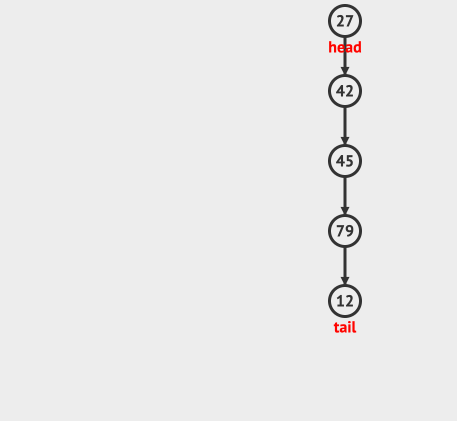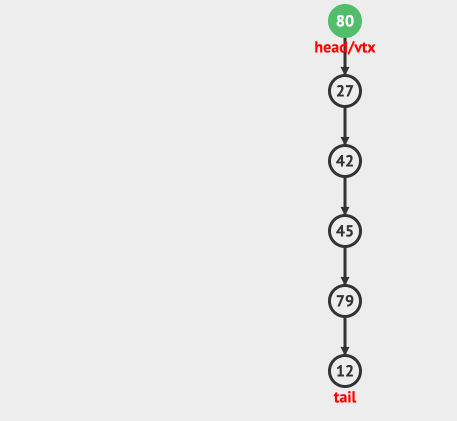# Look at the animation algorithm: stack stack

## Introduction

The stack should be a very simple and very useful data structure. The characteristic of the stack is FILO or LIFO.

In fact, the structure of many virtual machines is a stack. Because the stack is very effective in implementing function calls.

Today we will take a look at the structure and usage of the stack.

## The composition of the stack

A stack is an ordered linear list that can only be inserted or deleted at one end. This end is called the top end.

To define a stack, we need to implement two functions, one is push, which means push, and the other is pop, which means pop.

Of course, we can also define some other auxiliary functions, such as top: get the topmost node on the stack. isEmpty: Determine whether the stack is empty. isFull: Determine whether the stack is full or the like.

First look at the animation of the stack:Look at the animation of popping again:## Implementation of the stack

How is a stack with such a function implemented?

Generally speaking, the stack can be implemented with an array or a linked list.

### Use arrays to implement stacks

If an array is used to implement the stack, we can use the last node of the array as the head of the stack. In this way, only the last node in the array needs to be modified when operating the push and pop stacks.

We also need a topIndex to save the position of the last node.

The implementation code is as follows:

``````public class ArrayStack {

//实际存储数据的数组
private int[] array;
//stack的容量
private int capacity;
//stack头部指针的位置
private int topIndex;

public ArrayStack(int capacity){
this.capacity= capacity;
array = new int[capacity];
//默认情况下topIndex是-1，表示stack是空
topIndex=-1;
}

/**
* stack 是否为空
* @return
*/
public boolean isEmpty(){
}

/**
* stack 是否满了
* @return
*/
public boolean isFull(){
}

public void push(int data){
if(isFull()){
System.out.println("Stack已经满了，禁止插入");
}else{
array[++topIndex]=data;
}
}

public int pop(){
if(isEmpty()){
System.out.println("Stack是空的");
return -1;
}else{
return array[topIndex--];
}
}
}``````

### Use dynamic arrays to implement stacks

In the above example, our array size is fixed. In other words, the stack is limited in capacity.

What if we want to build a stack of unlimited capacity?

It's very simple. When pushing, if the stack is full, we can expand the underlying array.

The implementation code is as follows:

``````public void push(int data){
if(isFull()){
System.out.println("Stack已经满了，stack扩容");
expandStack();
}
array[++topIndex]=data;
}

//扩容stack，这里我们简单的使用倍增方式
private void expandStack(){
int[] expandedArray = new int[capacity* 2];
System.arraycopy(array,0, expandedArray,0, capacity);
capacity= capacity*2;
array= expandedArray;
}``````

Of course, there are many ways to expand the array, here we choose the multiplication method.

### Use linked list to achieve

In addition to using arrays, we can also use linked lists to create stacks.

When using a linked list, we only need to operate on the head node of the linked list. Insertion and deletion are the head nodes that are processed.

``````public class LinkedListStack {

class Node {
int data;
Node next;
//Node的构造函数
Node(int d) {
data = d;
}
}

public void push(int data){
}else{
Node newNode= new Node(data);
}
}

public int top(){
return -1;
}else{
}
}

public int pop(){
System.out.println("Stack是空的");
return -1;
}else{
return data;
}
}

public boolean isEmpty(){
}
}``````

learn-algorithm

The most popular interpretation, the most profound dry goods, the most concise tutorial, and many tips you don't know are waiting for you to discover!

Welcome to pay attention to my official account: "Program those things", know technology, know you better!##### 程序那些事
Spring,区块链,密码学,分布式,多线程等教程 欢迎关注我的公众号:程序那些事，更多精彩等着您！

813 声望
421 粉丝
0 条评论